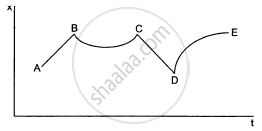# The Figure Shows the Displacement of a Particle Going Along The X-axis as a Function of Time. the Force Acting on the Particle is Zero in the Region - Physics

Short Note

The figure shows the displacement of a particle going along the X-axis as a function of time. The force acting on the particle is zero in the region(a) AB
(b) BC
(c) CD
(d) DE

#### Solution

(a) AB
(c) CD
Slope of the x-t graph gives velocity. In the regions AB and CD, slope or velocity is constant, i.e. acceleration is zero. Hence, from the second law, force is zero in these regions.

Concept: Newton’s Second Law of Motion
Is there an error in this question or solution?

#### APPEARS IN

HC Verma Class 11, Class 12 Concepts of Physics Vol. 1
Chapter 5 Newton's Laws of Motion
MCQ | Q 4 | Page 78